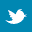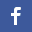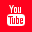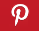Completely Solved C, C++ Programs Assignment.Quick Search Database Videos Tutorials Ebooks Forums FAQ Aboutus Household Industrial Manufacturing Service Shopping Transportation### C program to add, subtract and multiply two 2D matrix.

 Filed Under: C Assignments

PROBLEM STATEMENT:
Write a C program to add, subtract and multiply two 2D matrix.

THEORY:
In mathematics, a matrix is a rectangular array of numbers, such asAn item in a matrix is called an entry or an element. The example has entries 1, 9, 13, 20, 55, and 6. Entries are often denoted by a variable with two subscripts. Thus in the matrix above, a2,1 = 20. Matrices of the same size can be added and subtracted entry-wise and matrices of compatible sizes can be multiplied. These operations have many of the properties of ordinary arithmetic, except that matrix multiplication is not commutative, that is, AB and BA are not equal in general.

The sum A+B of two m-by-n matrices A and B is calculated entry-wise:
(A + B)i,j = Ai,j + Bi,j, where 1 ≤ i ≤ m and 1 ≤ j ≤ n.

The sum A+B of two m-by-n matrices A and B is calculated entry-wise:
(A - B)i,j = Ai,j - Bi,j, where 1 ≤ i ≤ m and 1 ≤ j ≤ n.

Multiplication of two matrices is defined only if the number of columns of the left matrix is the same as the number of rows of the right matrix. If A is an m-by-n matrix and B is an n-by-p matrix, then their matrix product AB is the m-by-p matrix whose entries are given by dot-product of the corresponding row of A and the corresponding column of B:

Matrix multiplication satisfies the rules (AB)C = A(BC) (associativity), and (A+B)C = AC+BC as well as C(A+B) = CA+CB (left and right distributivity).

ALGORITHM:
Begin
Begin function main
Read r1 //Row size of Array 1
Read c1 //Column size of Array 1
Read r2 //Row size of Array 2
Read c2 //Column size of Array 2

Allocate r1 and c1 to array a[ ][ ] and r2 and c2 to array b[ ][ ]

Print ’Enter elements of Matrix 1’
for i&#61663;0 to less than r1, increment i by 1
for j&#61663;0 to less than c1, increment j by 1
Read a[i][j]

Print ‘Enter elements of Matrix 2’
for i&#61663;0 to less than r2, increment i by 1
for j&#61663;0 to less than c2, increment j by 1
Read b[i][j]

Display Menu

do
Read ch
switch(ch)//Switching through the different functions according to choice
case 1:add(a,b,r1,c1,r2,c2)
break
case 2:sub(a,b,r1,c1,r2,c2)
break
case 3:mul(a,b,r1,c1,r2,c2)
break
case 4:Print ‘Terminating program’
break
if ch is not equal to any of the mentioned cases
Print ‘Wrong choice entered’
While ch is not equal to 4
End of function main

Begin function add(int **a,int **b,int r1,int c1,int r2,int c2)
if r1 is equal to r2 and c1 is equal to c2)
Print ‘The summation matrix is’
for i&#61663;0 to less than r1,increment i by 1
for j&#61663;0 to less than c1, increment j by 1
temp&#61663;a[i][j]+b[i][j]
Print temp
New Line
else
Print ‘The matrices cannot be added as we have different row and column no.s for the two matrices’
End function add

Begin function sub(int **a,int **b,int r1,int c1,int r2,int c2)
if r1 is equal to r2 and c1 is equal to c2)
Print ‘The difference matrix is’
for i&#61663;0 to less than r1,increment i by 1
for j&#61663;0 to less than c1, increment j by 1
temp&#61663;absolute value of (a[i][j]-b[i][j])
Print temp
New Line
else
Print ‘The difference cannot be found out as we have different row and column no.s for the two matrices’
End function sub

Begin function mul(int **a,int **b,int r1,int c1,int r2,int c2)
if c1 is equal to r2 //if row of 1st array and column of 2nd array is equal
for i&#61663;0 to less than r1,increment I by 1
for j&#61663;0 to less than c2, increment j by 1
sum&#61663;0
for k&#61663;0 to less than r2, increment k by 1 //loop to generate column of 1st and row of 2nd array
sum&#61663;sum+(a[i][k]*b[k][j])
Print sum
Newline
else
Print ’The matrices could not be multiplied because no. of columns in first matrix is not equal to no. of rows in second matrix’
End of function mul
End

PROGRAM LISTING:
//Program to add, subtract or multiply two 2D matrices.

#include<malloc.h>
#include<stdio.h>
#include<conio.h>
#include<math.h>

void main()
{
int **a,**b,r1,r2,c1,c2,i,j,ch;
//Function prototypes
void add(int **,int **,int,int,int,int);
void sub(int **,int **,int,int,int,int);
void mul(int **,int **,int,int,int,int);

//Accepting row and column sizes from user
printf("Enter row and column sizes of Matrix 1 n");
printf("Row ");
scanf("%d",&r1);
printf("Column ");
scanf("%d",&c1);
printf("Enter row and column sizes of Matrix 2 n");
printf("Row ");
scanf("%d",&r2);
printf("Column ");
scanf("%d",&c2);

//Allocating memory to the 2D arrays
a=(int **)malloc(r1*sizeof(int *));
for(i=0;i<r1;i++)
a[i]=(int *)malloc(c1*sizeof(int));

b=(int **)malloc(r2*sizeof(int *));
for(i=0;i<r2;i++)
b[i]=(int *)malloc(c2*sizeof(int));

//Accepting array elements
printf("Enter elements of Matrix 1n");

for(i=0;i<r1;i++)
for(j=0;j<c1;j++)
scanf("%d",&a[i][j]);

printf("Enter elements of Matrix 2n");

for(i=0;i<r2;i++)
for(j=0;j<c2;j++)
scanf("%d",&b[i][j]);

clrscr();

//Displaying inputted arrays
printf("Array 1 is : n");

for(i=0;i<r1;i++)
{
for(j=0;j<c1;j++)
printf("%d ",a[i][j]);
printf("n");
}//end of loop i

printf("Array 2 is : n");

for(i=0;i<r2;i++)
{
for(j=0;j<c2;j++)
printf("%d ",b[i][j]);
printf("n");
}//end of loop i

printf("n");
printf(" MENU n");
printf(" 1.ADDITION n");
printf(" 2.DIFFERENCE n");
printf(" 3.MULTIPLICATION n");
printf(" 4.EXIT n");
printf("n");

do
{
printf("n");
printf("Enter choice n");
scanf("%d",&ch);//Accepting users choice
switch(ch)//Switching through the different functions according to choice
{
case 1:add(a,b,r1,c1,r2,c2);
break;
case 2:sub(a,b,r1,c1,r2,c2);
break;
case 3:mul(a,b,r1,c1,r2,c2);
break;
case 4:printf("Terminating program n");
break;
default:printf("Wrong choice entered n");
}//end of switch
}//end of do
while(ch!=4);
}//end of main

void add(int **a,int **b,int r1,int c1,int r2,int c2)
{
int i,j,temp;
if(r1==r2 && c1==c2)//If row and column sizes of both arrays match
{
printf("The summation matrix is :n");
for(i=0;i<r1;i++)
{
for(j=0;j<c1;j++)
{
temp=a[i][j]+b[i][j];
printf("%d ",temp);
}//end of loop j
printf("n");
}//end of loop i
}//end of if
else
printf("The matrices cannot be added as we have different row and column no.s for the two matrices n");
}//end of add

void sub(int **a,int **b,int r1,int c1,int r2,int c2)
{
int i,j,temp;
if(r1==r2 && c1==c2)//If row and column sizes of both arrays match
{
printf("The difference matrix is :n");
for(i=0;i<r1;i++)
{
for(j=0;j<c1;j++)
{
temp=abs(a[i][j]-b[i][j]);
printf("%d ",temp);
}//end of loop j
printf("n");
}//end of loop i
}//end of if
else
printf("The difference cannot be found as we have different row and column no.s for the two matrices n");
}//end of sub

void mul(int **a,int **b,int r1,int c1,int r2,int c2)
{
int i,j,k,sum;
if(c1==r2)//if row of 1st and column of 2nd array is equal
{
for(i=0;i<r1;i++)
{
for(j=0;j<c2;j++)
{
sum=0;
for(k=0;k<r2;k++)//loop to generate column of 1st and row of 2nd array
sum=sum+(a[i][k]*b[k][j]);
printf("%d ",sum);
}//end of loop j
printf("n");
}//end of loop i
}//end of if
else
printf("The matrices could not be multiplied because no. of columns in first matrix is not equal to no of rows in second matrix");
}//end of mul

OUTPUT:
Enter row and column sizes of Matrix 1
Row 3
Column 3
Enter row and column sizes of Matrix 2
Row 3
Column 3
Enter elements of Matrix 1
1
2
3
4
5
6
7
8
9
Enter elements of Matrix 2
1
2
3
4
5
6
7
8
9

Array 1 is :
1 2 3
4 5 6
7 8 9
Array 2 is :
1 2 3
4 5 6
7 8 9

MENU
1.ADDITION
2.DIFFERENCE
3.MULTIPLICATION
4.EXIT

Enter choice
1
The summation matrix is :
2 4 6
8 10 12
14 16 18

Enter choice
2
The difference matrix is :
0 0 0
0 0 0
0 0 0

Enter choice
3
30 36 42
66 81 96
102 126 150

Enter choice
4
Terminating program

DISCUSSION:
Matrix addition and subtraction can only be performed if the row and columns sizes of the two matrices are equal.
Matrix multiplication is not commutative. The size of the column of the first matrix should be equal to the size of the row of the second matrix.
Function prototypes should be defined before declaration of the functions
Keep a check for choice entered not in the list of options in the menu.Back to main directory:Software Practical

 @import url(http://www.google.com/cse/api/branding.css);